### Home > PC > Chapter 1 > Lesson 1.3.1 > Problem1-103

1-103.
1. Find the inverse of each function below. Use correct notation. Homework Help ✎

1. f(x) =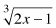2. g(x) =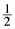(x − 3) + 1

3. h(x) = 2x3/2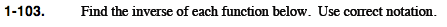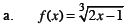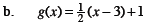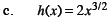Substitute y for the function notation.

Switch every x for y and every y for x.

Solve for y. This is the inverse function.